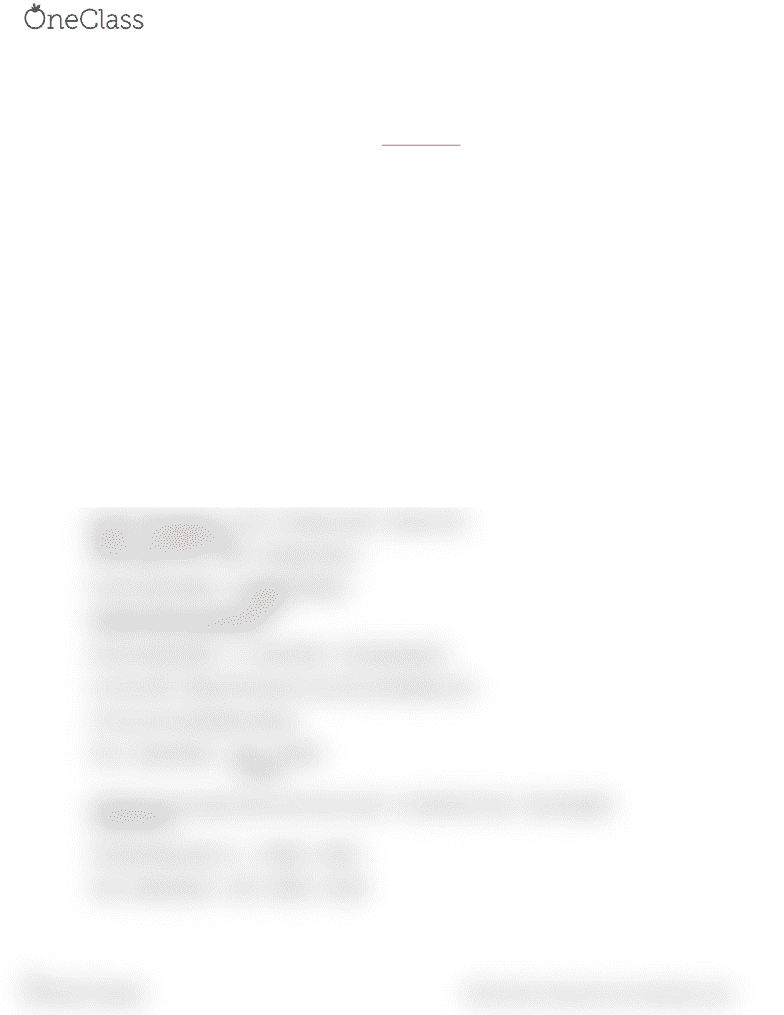Class Notes (1,100,000)
CA (650,000)
McMaster (50,000)
COMMERCE (2,000)
Lecture 23

# COMMERCE 1AA3 Lecture 23: Class 23

Department
Commerce
Course Code
COMMERCE 1AA3
Professor
Lecture
23

This preview shows half of the first page. to view the full 2 pages of the document.Financial Accounting - COMMERCE 1AA3: Class 23
Weighted Average Cost changes every time a PURCHASE is made (not a sale).
WAC = COGAS/Units available for sale
How to decide when an expense is Capitalized vs Expensed: !
If the beneﬁt will extend past the current reporting period, then it should be capitalized. However
if the beneﬁt lasts only for this reporting term, then it should immediately be expensed.
Capital expenditures have a future beneﬁt.
Revenue expenditures end up in the income statement as an expense in this period. They
reduce income and hence they reduce tax immediately.
Capital expenditures end up in the balance sheet. They are brought into the income statement
over time in the form of depreciation expense. Thus they reduce income over time and therefore
income tax is higher in earlier years.
—————
*** LOOK AT THE SLIDE ABOUT ANNUAL DEPRECIATION EXPENSE***
Straight Line Depreciation
Annual Depreciation = (Cost - Salvage Value) / (Useful Life)
Rate = 1 / (Useful Life)
Amortizable Cost = (Cost - Salvage Value)
Annual Depreciation = (100000-20000)/5
= 16000
Double Declining Balance
Annual Depreciation = 2 / (Useful life) * Carrying balance
It is the ONLY method that does not use the amortizable cost.
It is the only accelerated method.
Year 1 Depreciation = (2/5) * 100000
= 40000
Therefore the carrying value at the end of year 1 is (Historical Cost) - (Accumulated
Depreciation)
Carrying Value after yr 1 = 100000 - 40000
Year 2 depreciation = (2/5) * 60000 = 24000
find more resources at oneclass.com
find more resources at oneclass.com